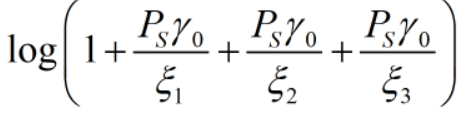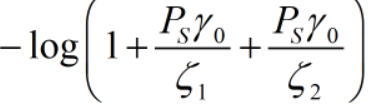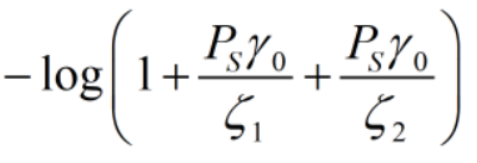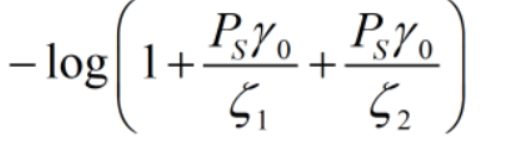# How to solve the objective function in images with cvx?

How to solve the objective function in images with cvx?in the objective function can be entered into CVX using a first-order Taylor expansion.is a concave function that is fed into CVX and keeps reporting the error log(convex ).
How to equivalently translate the objective function to the type recognized by CVX? Can you give me the cvx statement after the equivalent conversion? Thanks a lot?

It looks like the objective and the last two constraints are non-convex.

The objective consists of `{log-sum-inv} - {log-sum-inv}`, which is`{convex} - {convex}`. There are plenty of posts on this forum for how to deal with `log-sum-inv`. But the first term is a convex term which is being maximized, which is a non-convex operation. So that makes this a non-convex problem, unless somehow the input data is such that it allows a simplification into a concave objective (being maximized).

The last two constraints appear to have quadratic functions of a variable on the wrong side of the inequality to be convex.

Dear Mark_L_Stone,
I agree with your point about the first term of the objective function being a convex function, but that is I can use the first order Taylor expansion to approximate that expression as a linear function with respect to the optimization variables, and the transformed linear function can be considered as a concave function in the domain of definition that can be maximized.
The functionis convex.
The functionis concave,due to the “-”.
According to the convexity-preserving operation, sinceis a concave function,add a linear function must be a concave function, and it can be maximized.
My question now is how to use cvx to expressequivalently.

You can search on this forum for `log-sum-inv` to see how to handle that.

That still doesn’t address the non-convex constraints.